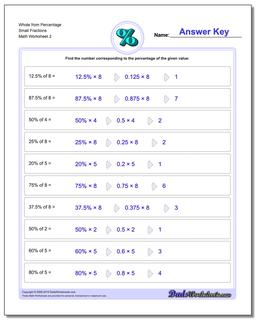# Math Worksheets: Percentages: Percentages: Whole from Percentage Small Fractions (Second Worksheet)## Whole from Percentage Small Fractions (Second Worksheet)

PropertyValue
DescriptionWhole from Percentage Small Fractions: Percent worksheets where students are given a whole number and a percentage, and must calculate the fraction of the whole represented. (Second Worksheet)
Resource TypeWorksheet